# 数据分析项目：用户消费行为分析

### 数据分析项目：用户消费行为分析

##### 数据字段：
• user_id 用户ID
• order_dt: 购买日期
• order_products: 购买产品数
• order_amount: 购买金额

0：数据准备阶段（数据预处理）
1：按月数据分析
2：用户个体消费分析
3：用户消费行为分析
4：复购率，回购率分析


#### 0: 数据准备

0.1首先导入常用的python库

import pandas as pd
import numpy as np
import matplotlib.pyplot as plt
%matplotlib inline
import seaborn as sns
plt.rcParams['font.sans-serif']=['SimHei'] #用来正常显示中文标签


0.2 读取数据，观察数据

1 columns = ['user_id','order_dt','order_products','order_amount']
# 查看数据
# 大概格式了解
data.info（）
# 数据字段
user_ud 用户ID
order_dt: 购买日期
order_products: 购买产品数
order_amount: 购买金额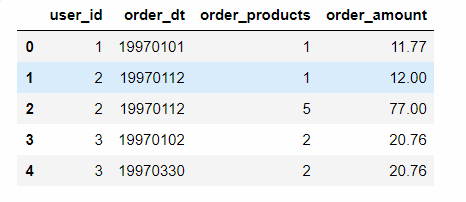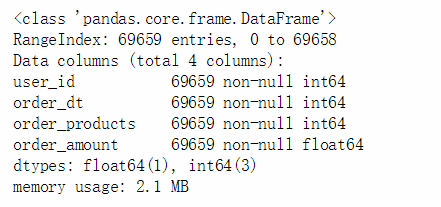data['order_dt'] = pd.to_datetime(data.order_dt,format='%Y%m%d')
# 新增一个月 （对月进行分析） values（数组形式） 因为很多是进行月份的一个处理
data['month'] = data.order_dt.values.astype('datetime64[M]')
# 处理后，查看数据，此时数据时间的格式
data.info()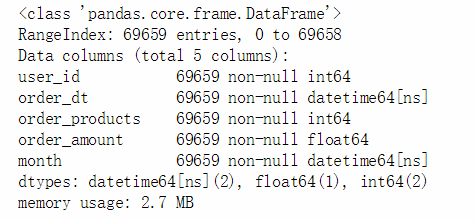# describe是描述统计
data.describe()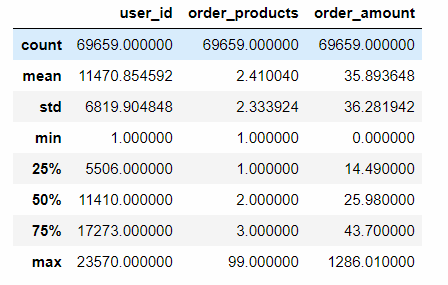• 观察数据，用户平均订单必输2.4个商品，标准差为2.3，略有波动，中位数书为2个商品，说明绝大部分订单的购买量不多，最大值为99，有一定的极值干扰
• 用户消费均值35元左右，中位数25元，最大金额达到了1286元，有一定极值干扰

#### 1:按月数据分析

• 每月消费总金额分析
• 每月消费次数
• 每月产品购买量
• 每月消费人数
# 按月分组
grouped_month = data.groupby('month')

fig = plt.figure(figsize = (8,12))
# 按月金额数
ax1.plot(grouped_month.order_amount.sum(),c='blue')
ax1.set_title('每月消费总金额变化趋势')
# 按月的订单数
ax2.plot(grouped_month.user_id.count(),c='blue')
ax2.set_title('每月订单数变化趋势')
# 按月产品数
ax3.plot(grouped_month.order_products.sum(),c='blue')
ax3.set_title('每月消费产品购买量')
# 每月用户人数  这里需要去重，有些用户重复购买
ax4.plot(grouped_month.user_id.apply(lambda x:len(x.drop_duplicates())),c='blue')
ax4.set_title('每月用户数变化趋势')

• 按月进行统计，下面通过折线图分别查看数据变化趋势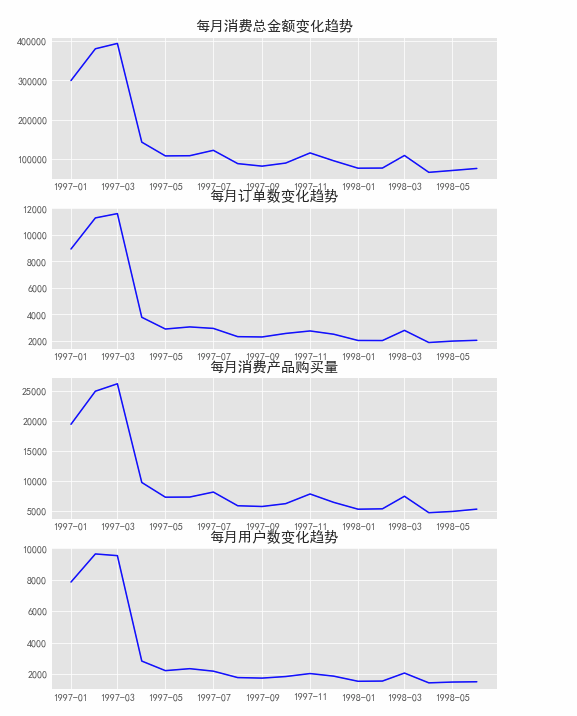##### 数据透视查看，按月根据用户购买金额，订单数，用户人数
data.pivot_table(index='month',
values = ['order_products','order_amount','user_id'],
aggfunc = {'order_products':'sum',
'order_amount':'sum',
'user_id':'count'})


#### 2 用户个体消费分析

1. 用户消费金额，消费次数描述统计
2. 用户消费金额和消费次数散点图
3. 用户消费金额的分布图
4. 用户消费次数的分布图
5. 用户累计消费金额占比（百分之多少的用户占了百分之多少的消费额）

1 用户消费金额和消费次数描述统计

grouped_user = data.groupby('user_id')
grouped_user.sum().describe()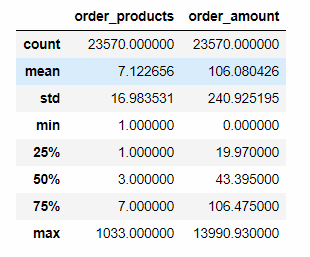• 用户平均购买量7张CD,标准差17，波动比较大，但是中位值只有3，说明小部分用户购买了大量的CD
• 用户平均消费为106元，中位值为43，也有极值干扰
• 消费一般都会符合二八法则

2 用户消费金额和消费次数散点图

# 设置排除一些极值的干扰进行观察
grouped_user.sum().query('order_amount < 4000').plot.scatter(x='order_amount',y='order_products')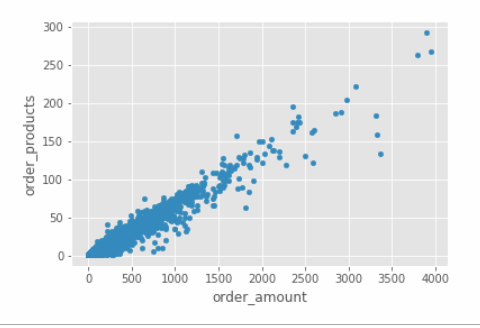• 绘制散点图，由于产品比较单一，购买金额和产品数呈线性关系

03-用户消费金额的分布图（二八法则）

grouped_user.sum().order_amount.plot.hist(bins=20)
# bins=20，就是分成20块，最高金额是14000，每个项就是700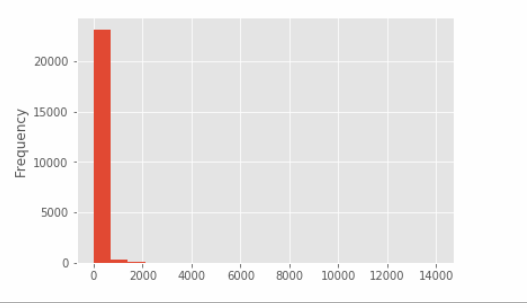• 由直方图可知，用户消费金额，大部分消费金额能力确实不高，绝大部分呈几种在很低的消费档次，高消费用户在图中基本看不到，这也符合消费行为的行业规律
• 小部分异常值干扰判断，可以过滤操作排除异常

04 用户消费次数分布图

grouped_user.sum().query('order_products<92').order_products.hist(bins = 40)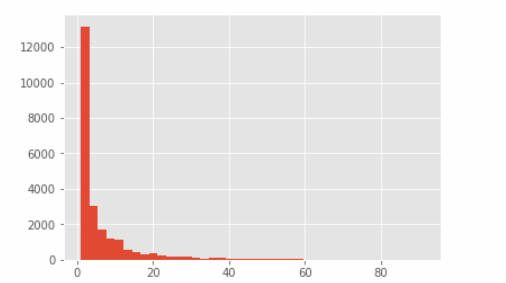• 使用切比雪夫定理过滤异常值，计算95%的数据分布情况 （均值+5*标准差）

05 用户累计消费金额占比

user_cumsum = grouped_user.sum().sort_values('order_amount').apply(lambda x: x.cumsum() / x.sum())
user_cumsum.reset_index().order_amount.plot()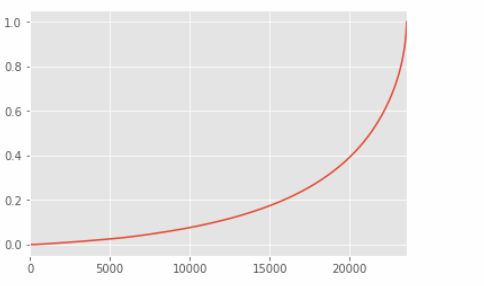• 通过观察，50%的用户仅仅贡献了15%的消费额度，而排名前五千用户就贡献了%60的消费额
print(user_cumsum.head(8))
print(user_cumsum.tail(8))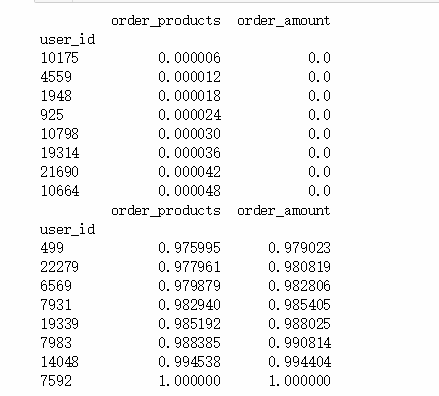• 上面是一些累积占比的数据，数据中存在金额为0，可能是一些活动赠送等原因

#### 3. 用户消费行为分析

1. 用户第一次消费（首购）
2. 用户最后一次消费
3. 新老客户消费比
• 多少用户仅仅消费一次
• 每月新客占比
4. 用户分层
• RFM模型
• 新、老、活跃、回流/不活跃
5. 用户购买周期（按订单）
• 用户消费周期描述
• 用户消费周期分布
6. 用户生命周期（按第一次 & 最后一次消费）
• 用户生命周期描述
• 用户生命周期分布
##### 1 第一次购买时间分布
# min()  时间最小，第一次购买
grouped_user.min().order_dt.value_counts().plot()
# 2月发生较大下跌  渠道发生变化，或者其他  可以做一些假设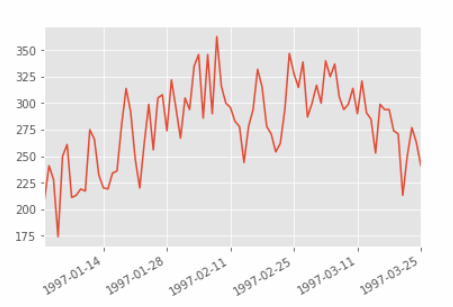• 用户第一次购买分布，集中在前三个月，其中2月11日至2月25日有一次剧烈波动
##### 02 最后一次购买时间
grouped_user.max().order_dt.value_counts().plot()

• 用户最后一次购买的分布比第一次购买分布广
• 大部分最后一次购买在前三个月，说明很多用户购买一次后就不再进行购买
• 随着时间递增，最后一次购买数在递增，消费呈线性流失上升的情况
##### 03 新老客户消费比
# 用户第一次购买与最后一次购买
user_life =grouped_user.order_dt.agg(['min','max'])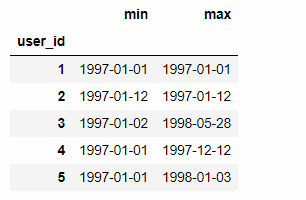(user_life['min'] == user_life['max']).value_counts()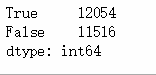rate = (user_life['min'] == user_life['max']).value_counts()
labels = ['只消费一次用户','多次消费用户']
plt.axis('equal') # 保证长宽相等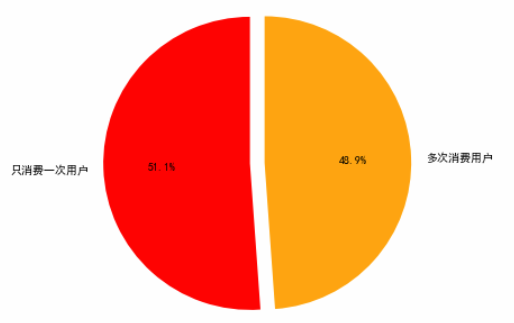• True 第一次购买时间和最后一次购买时间一样，有一般用户只消费了一次
##### 04 用户分层
#  RFM  最近一次消费  次数  消费额  进行透视
rfm = data.pivot_table(index = 'user_id',
values = ['order_products','order_amount','order_dt'],
aggfunc = {'order_dt':'max',
'order_amount':'sum',
'order_products':'sum'})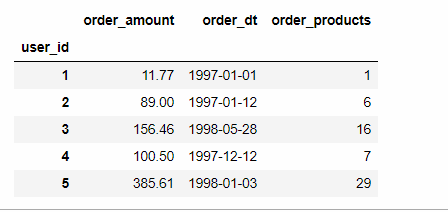# 表示离最近一次消费的时间间隔
# 数值越大，离现在的时间越长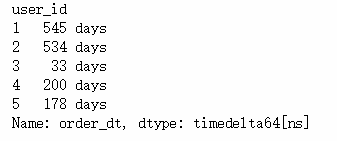# /np.timedelta64(1,'D')  消除单位   进行重命名
rfm['R'] = -(rfm.order_dt - rfm.order_dt.max()) / np.timedelta64(1,'D')
rfm.rename(columns = {'order_products':'F','order_amount':'M'},inplace=True)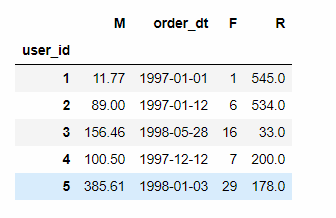rfm[['R','F','M']].apply(lambda x:x-x.mean()).head()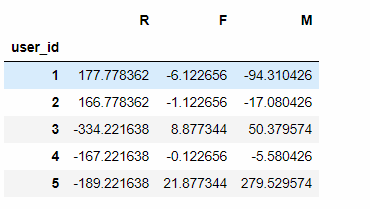• 数据解读： 负数是小于的 正式大于的
• R：离最近一次购买的天数 F：产品数（消费次数） M：消费金额 与均值的对比
def rfm_func(x):
level = x.apply(lambda x:'1' if x>= 0 else '0')
# 字符串拼接
# 111，R>0,是距离平均消费时间要久，R越大 说明没有消费时间越久  ，F >0 M>0,消费次数和金额也是较高的，重要价值客户，依次类推
label = level.R + level.F + level.M
d = {
'111':'重要价值客户',
'011':'重要保持客户',
'101':'重要挽留客户',
'001':'重要发展客户',
'110':'一般价值客户',
'010':'一般保持客户',
'100':'一般挽留客户',
'000':'一般发展客户'
}
result = d[label]
return result
# x - x.mean() （具体真实情况可以修改，不一定需要用均值）   切比雪夫也可以 > 200 极值人工处理掉
rfm['label'] = rfm[['R','F','M']].apply(lambda x:x-x.mean()).apply(rfm_func,axis=1)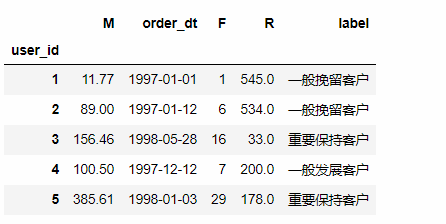• 数据解读： 1 时间比较长，只消费了一次，消费金额低 所以是 一般挽留客户
# 统计查看
rfm.groupby('label').sum()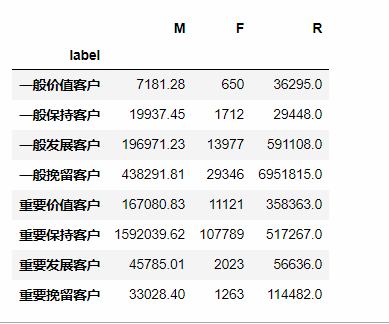• 重要保持客户贡献了159万左右 等等
rfm.groupby('label').count()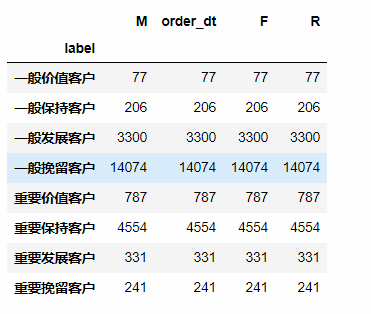# 各类类型用户占比
use_c = rfm.groupby('label').count()
plt.axis('equal')
labels = ['一般价值客户','一般保持客户','一般发展客户','一般挽留客户','重要价值客户','重要保持客户','重要发展客户','重要挽留客户']
plt.pie(use_c['M'],
autopct='%3.1f%%',
labels = labels,
pctdistance=0.9,
labeldistance = 1.2,
startangle = 15)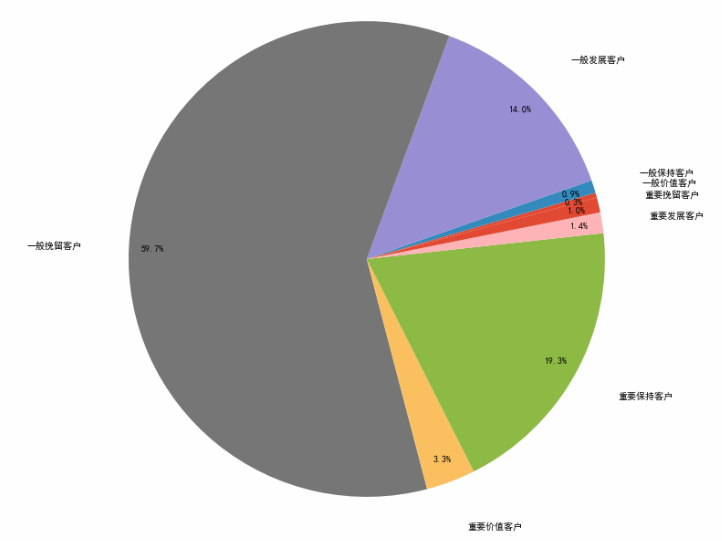# 对应标签，使用不同颜色表示
# 绿色为重要价值客户，红色为非重要价值用户
rfm.loc[rfm.label == '重要价值客户','color'] = 'g'
rfm.loc[~(rfm.label == '重要价值客户'),'color'] = 'r'
rfm.plot.scatter('F','R',c=rfm.color)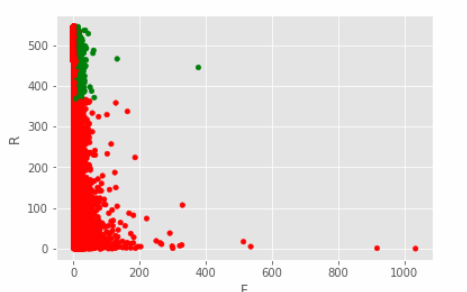rfm.head()
# rfm 象限法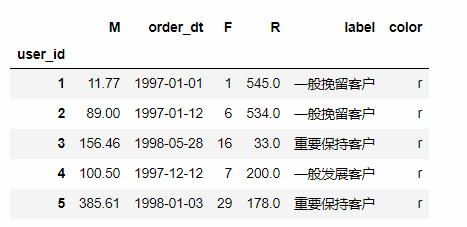rfm.groupby('label').sum()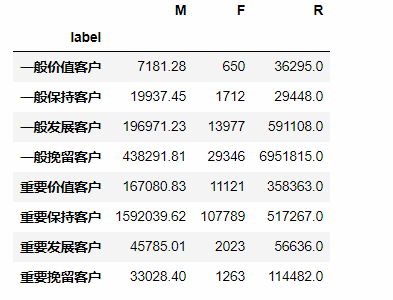• 尽量用小部分的用户覆盖大部分的额度
• 不要为了数据好看划分等级
• 极值会拉均值
• 根据数据可以和业务相结合，如何提升一些重要的指标
##### 05 用户购买周期
pivoted_counts = data.pivot_table(index = 'user_id',
columns = 'month',
values = 'order_dt',
aggfunc = 'count').fillna(0)
# 按月份进行对比，1月份哪些是购买的，再去对比二月份哪些是购买的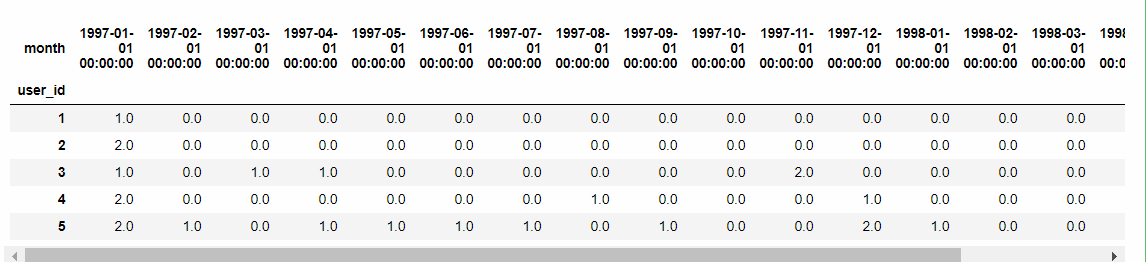消费过的为1 ，没消费过的为0
data_purchase = pivoted_counts.applymap(lambda x: 1 if x > 0 else 0)
data_purchase.tail()
# 透视会补上  第一次从3月分开始购买 前面补成0 ， 需要进行判，第一次消费作为生命周期的起始
# se.columns.values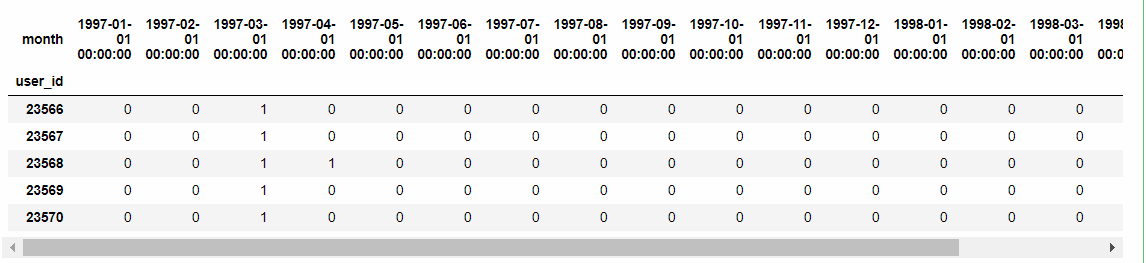def active_status(data):
status = []
for i in range(18):

#若本月没有消费
if data[i] == 0:
if len(status) > 0:
if status[i-1] == 'unreg':
status.append('unreg')
else:
status.append('unactive')
else:
status.append('unreg')
#若本月消费
else:
if len(status) == 0:
status.append('new')
else:
if status[i-1] == 'unactive':
status.append('return')
elif status[i-1] == 'unreg':
status.append('new')
else:
status.append('active')
# 这里需要对返回的值进行转换，将列表转为Series
return pd.Series(status, index = pivoted_counts.columns)

• 若本月没有消费
• 若之前是未注册，则依旧未注册
• 若之前有消费，则为流失/不活跃
• 其他情况，为未注册
• 若本月有消费
• 若是第一次消费，则为新用户
• 如果之前有过消费，则上个月为不活跃，则为回流
• 如果上个月为未注册，则为新用户
• 除此之外，为活跃
purchase_stats = data_purchase.apply(active_status,axis=1,result_type ='expand')
# df.rename(columns={ df.columns: "new name" }, inplace=True)
# purchase_stats.rename(columns={purchase_stats.columns:data_purchase.columns})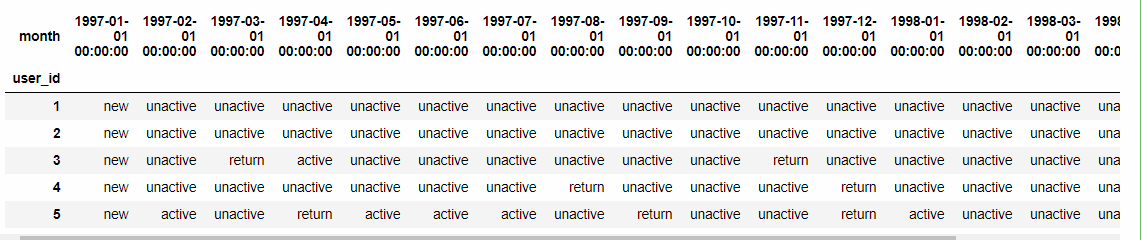purchase_status_ct = purchase_stats.replace('unreg',np.NaN).apply(lambda x : pd.value_counts(x))
purchase_status_ct
# 未注册不希望参与处理  设置为空值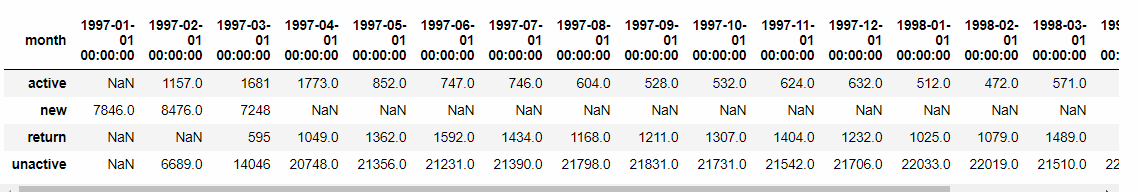# 各个状态的占比
purchase_status_ct.fillna(0).T.apply(lambda x:x/x.sum(),axis=1)
purchase_status_ct.fillna(0).T.plot.area()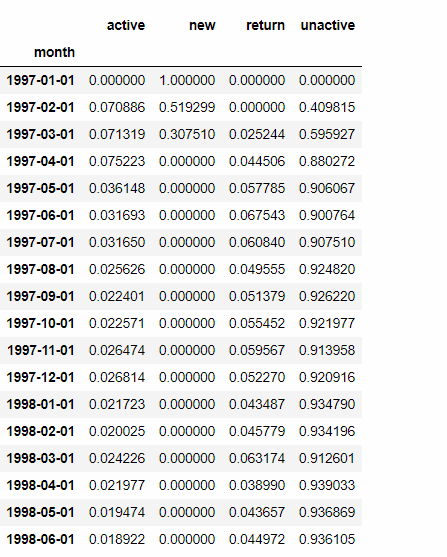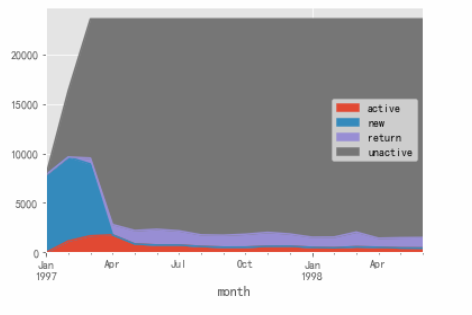• 活跃用户，持续消费的用户，对应的使消费运营质量
• 回流用户，之前不消费本月才消费，对应的使唤回运营
• 不活跃用户，对应的是流失

06 用户购买周期

data.order_dt.head(10)

# 把所有的数据进行一个错位
DataFrame.
shift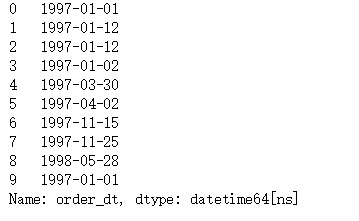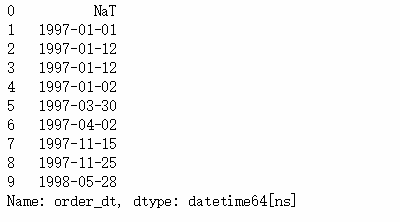#   一个用户可能多个订单  每个订单的间隔
order_diff = grouped_user.apply(lambda x:x.order_dt - x.order_dt.shift())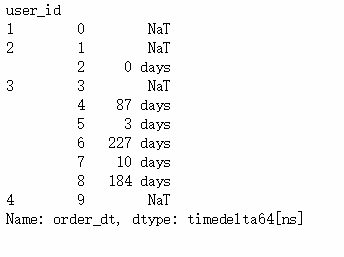ID为3的用户 3-4间隔了87天

NaT表示一个订单

order_diff.describe()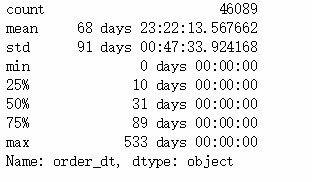• 每个用户的平均订单是68天 中位值31天 最大值533天
# 去除单位  间隔天数分布图
(order_diff / np.timedelta64(1,'D')).hist(bins=20)
order_diff / np.timedelta64(1,'D')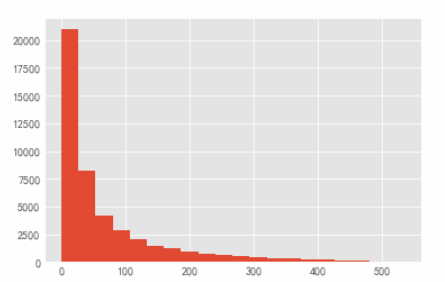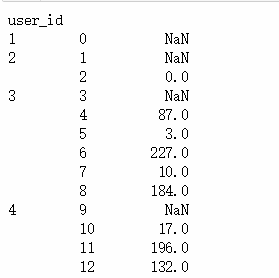(user_life['max'] - user_life['min']).describe()
# 整体的生命周期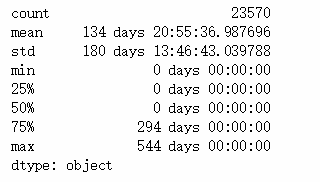((user_life['max'] - user_life['min']) / np.timedelta64(1,'D')).hist(bins = 40)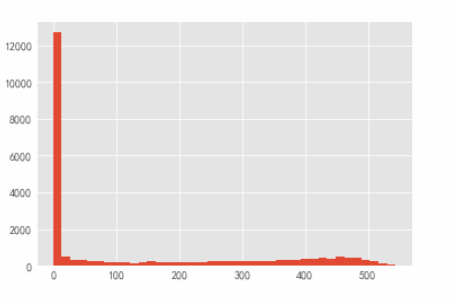• 用户的生命周期受只购买一次的用户影响比较厉害 （可以进行排除 当特殊情况，想提取出购买一次以上的用户）
• 用户均消费134天，中位数仅为0
u_l = ((user_life['max'] - user_life['min']).reset_index() / np.timedelta64(1,'D'))
u_l[u_l > 0].hist(bins=40)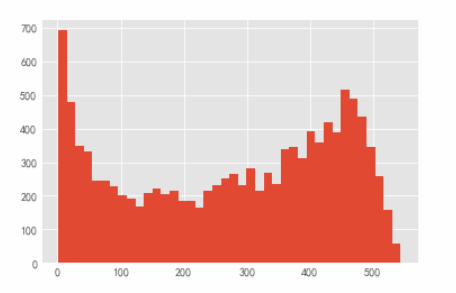• 左边比较高，还是有些用户的生命周期是比较短的，例如10、20天等
• 有不少用户生命周期也是比较稳定

#### 4.复购率和回购率分析

• 复购率
• 自然月内，购买多次的用户占比
• 回购率
• 曾今购买过的用户在某一时期内的再次购买占比
pivoted_counts.head()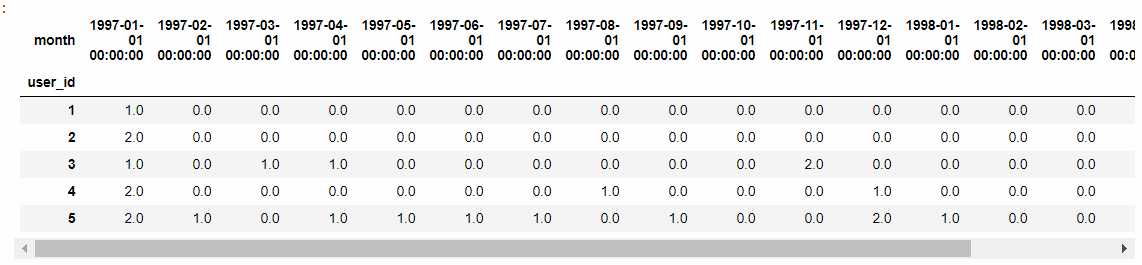# 购买大于1次的 赋值为1 ，然后小于等于1 的 如果是购买次数是0，则赋值为空，否则 就是购买一次，赋值为0
purchase_r = pivoted_counts.applymap(lambda x: 1 if x > 1 else np.NaN if x == 0 else 0)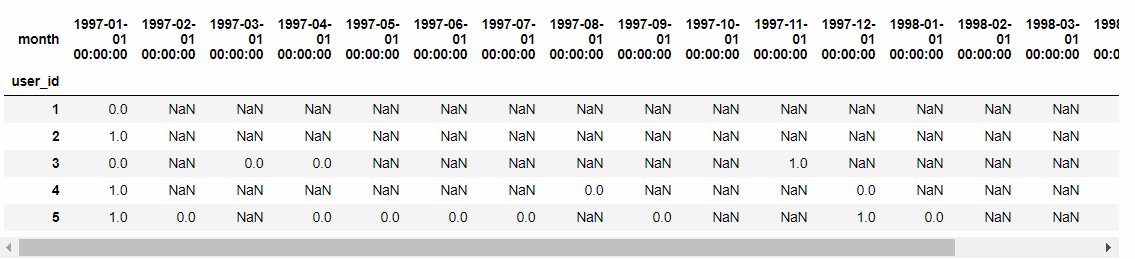# sum（） 0 不计数，count（） 0计数
(purchase_r.sum() / purchase_r.count()).plot(figsize = (10,4))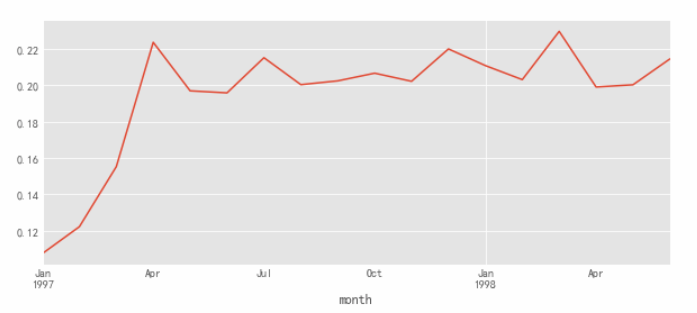• 复购率稳定在20% 左右，前三个月因为有大量新用户涌入，而这些用户只购买了一次，所以导致复购率较低
# 回购率 只需要0  1 代表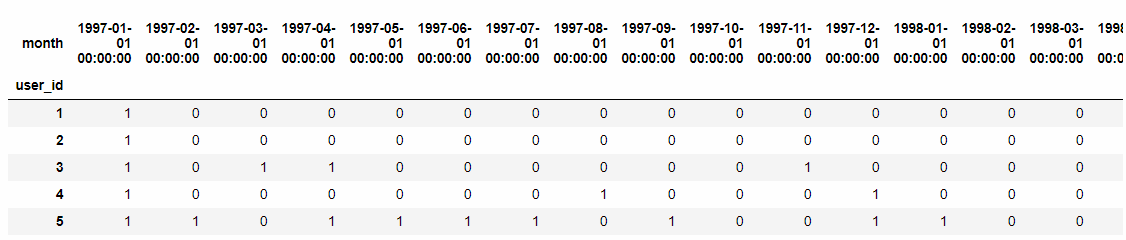def purchase_back(data):
status = []
for i in range(17):
if data[i] == 1: # 本月进行过消费
if data[i+1] == 1: # 下一月是否进行消费
status.append(1) #消费为1 回购了
if data[i+1] == 0:
status.append(0) # 未消费则为0 没有回购
else:
status.append(np.NaN) # 之前没消费则不计
status.append(np.NaN) # 最后一个月没有判断需要补上
return pd.Series(status,data_purchase.columns)
purchase_b = data_purchase.apply(purchase_back, axis =1)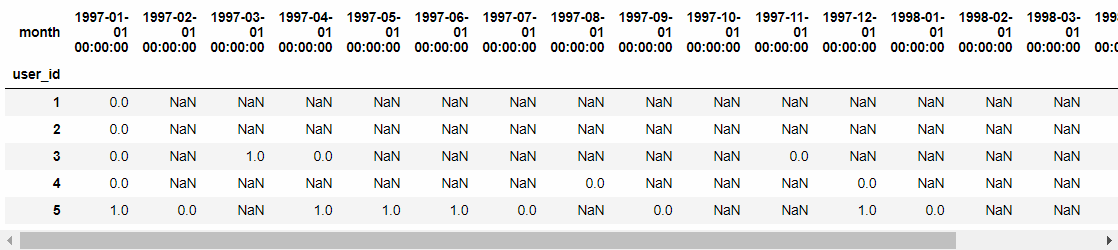• 0 表示上个月购买了，下个月没有进行消费，则是没有回购 ，
• 1代表当月消费过次月依旧消费，表示回购了
• NAN表示当月没有消费（不进行计算）
# sum() 0 1进行求和，表示次月消费过的
(purchase_b.sum() / purchase_b.count()).plot(figsize = (10, 4))• 回购率在30%左右波动，比复购率要高，综合分析得出，新客整体质量低于老客，老客忠诚度（回购率）表现较好，消费频次稍次，这个是是CDNow网站的用户消费特征
posted @ 2019-05-03 19:02  东西  Views(236)  Comments(0Edit  收藏